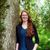# QlikView App Dev

Discussion Board for collaboration related to QlikView App Development.

Announcements
Our next Qlik Insider session will cover new key capabilities. Join us August 11th REGISTER TODAY
cancel
Showing results for
Did you mean:Not applicable

## expression

Hi

Appreciate any help. We have built the following expression in our script which provides us with a distance in miles from a certain postcode:

ACOS(SIN([Latitude]*Pi()/180)*SIN([M27 Lat]*Pi()/180)+COS([Latitude]*Pi()/180)*COS([M27 Lat]*Pi()/180)*COS(([M27 Long]*Pi()/180)-([Longitude]*Pi()/180)))*6371 as [Distance from M27(km)],
ACOS(SIN([Latitude]*Pi()/180)*SIN([M27 Lat]*Pi()/180)+COS([Latitude]*Pi()/180)*COS([M27 Lat]*Pi()/180)*COS(([M27 Long]*Pi()/180)-([Longitude]*Pi()/180)))*3959 as [Distance from M27(miles)]

This works as you can see on the attached (refer to list box 'Distance from M27(miles)). However, as this brings though a long list of distances we would like to merge these into categories (0.01 - 1 miles, 1.01 - 2 miles, 2.01 -3 miles, 3 miles +). Normally a expression like below does the trick; however, as 'Distance from M27miles isnt a field in the data (its a calculated one) doesn't seem to like it- get error when reloading. So I tried building the expression in the list box; however, doesn't seem to work.

Can anyone help?

=IF [Distance from M27(miles)]>=1.01 and [Distance from M27(miles)] <=2,'1-2 miles',
IF (
[Distance from M27(miles)]>=2.01 and [Distance from M27(miles)] <=3,'2-3 miles',
IF (
[Distance from M27(miles)]>=3.01 and [Distance from M27(miles)] <=10,'3 miles +')))

1 Solution

Accepted SolutionsMVP

Used an expression for the field on the list box and took the expression off.

9 RepliesMVP

Is this what you're looking for?Not applicable
Author

Hi

Yes that looks great but can you confirm what you have done?

Cheers

ChrisPartner

Hi,

I tried to fix the sorting issue in the field using "Dual()". Perhaps this his helpful for someone.By the way I would add this line in script as preceding load. (I commented it out because I couldn't test it) Than add a listbox for this field.

Best Regards,

PeterMVP

Used an expression for the field on the list box and took the expression off.Not applicable
Author

Hi peschu82, Nicole Smith, and anybody else who can help.

Probably being cheeky but wondering if this grouped box with the different distances could be developed so whichever of the 5 postcodes you pick it works. I know its trivial but if it worked it would save needing 5 boxes. Please refer to atatched and boxes entitled ' grouped ' and post code overall'

I tried extending expression in a list box and preceeding load ins cript but doesnt seem to work. I know this because I have created  a box for M28 in addition to one for M27 and they produce different results.

IF ([Distance from M27(miles)]>=0.01 and [Distance from M27(miles)] <=1,'1 mile or less',
IF ([Distance from M27(miles)]>=1.01 and [Distance from M27(miles)] <=2,'1-2 miles',
IF ([Distance from M27(miles)]>=2.01 and [Distance from M27(miles)] <=3,'2-3 miles',
IF ([Distance from M27(miles)]>=3.01 and [Distance from M27(miles)] <=10,'3 miles +',
IF ([Distance from M28(miles)]>=0.01 and [Distance from M28(miles)] <=1,'1 mile or less',
IF ([Distance from M28(miles)]>=1.01 and [Distance from M28(miles)] <=2,'1-2 miles',
IF ([Distance from M28(miles)]>=2.01 and [Distance from M28(miles)] <=3,'2-3 miles',
IF ([Distance from M28(miles)]>=3.01 and [Distance from M28(miles)] <=10,'3 miles +'))))))))

Cheers

ChrisPartner

Hi Chris,

I have question if I understood right...

You want to avoid, that you must create a listbox for every item in "Post Code Over" (m27, m28...)? iN Other words you just want one listbox for the distances like 1-2 Miles and one listbox for the post codes?

And should it be possible to select multiple post codes at the same time?

PeterNot applicable
Author

Hi,

Yes exactly. Apologies..hard to explain sometimes!

Mulitple postcodes at the same time is a bonus but not required.

Cheers

ChrisMVP

Try this:

=IF (([Distance from M27(miles)]>=0.01 and [Distance from M27(miles)] <=1) or ([Distance from M28(miles)]>=0.01 and [Distance from M28(miles)]<=1),'1 mile or less',

IF (([Distance from M27(miles)]>=1.01 and [Distance from M27(miles)] <=2) or ([Distance from M28(miles)]>=1.01 and [Distance from M28(miles)] <=2),'1-2 miles',

IF (([Distance from M27(miles)]>=2.01 and [Distance from M27(miles)] <=3) or ([Distance from M28(miles)]>=2.01 and [Distance from M28(miles)]<=3),'2-3 miles',

IF (([Distance from M27(miles)]>=3.01 and [Distance from M27(miles)] <=10) or ([Distance from M28(miles)]>=3.01 and [Distance from M28(miles)] <=10),'3 miles +'))))Partner

I tried to add 2 variables:

1.  to get the selected postcode: vPostcode = GetFieldSelections([Post Code overall])

2. vDistance = '[Distance from '& '\$(vPostcode)' & '(miles)]'

3. I tried to replace the IF expression in the Listbox with:

=IF(\$(vDistance) >= 0.01 and \$(vDistance) <= 1,'1 mile or less',

IF(\$(vDistance) >= 1.01 and \$(vDistance) <= 2,'1-2 miles',

IF(\$(vDistance) >= 2.01 and \$(vDistance) <= 3,'2-3 miles',

IF(\$(vDistance) >= 3.01 and \$(vDistance) <=10,'3 miles +'))))

The behavior is a bit strange... but perhaps you can play around with it.Community Browser